# CBSE Solutions for Class 10 English

#### GSEB std 10 science solution for Gujarati check Subject Chapters Wise::

If the sum of zeroes of the quadratic polynomial 3x2 – kx + 6 is 3, then find the value of k. (2012)

જવાબ : Here p = 3, q = -k, r = 6
Sum of the zeroes, (α + β) = -q/p= 3 …..(given)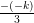= 3
k = 9

If α and β are the zeroes of the polynomial ay2 + by + c, find the value of α2 + β2.

જવાબ :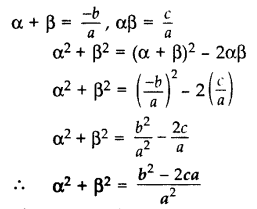If the sum of the zeroes of the polynomial p(x) = (k– 14) x2 – 2x – 12 is 1, then find the value of k. (2017 D)

જવાબ : p(x) = (k2 – 14) x2 – 2x – 12
Here a = k2 – 14, b = -2, c = -12
Sum of the zeroes, (α + β) = 1 …[Given in question]
⇒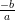= 1
⇒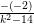= 1
⇒ k2 – 14 = 2
⇒ k2 = 16
⇒ k = ±4

If α and β are the zeroes of a polynomial such that α + β = -6 and αβ = 5, then find the polynomial. (2016 D)

જવાબ : Quadratic polynomial is x2 – (Sum)x + (Product) = 0
⇒ x2 – (-6)x + 5 = 0
⇒ x2 + 6x + 5 = 0

Question 5.
A quadratic polynomial, whose zeroes are -4 and -5, is …. (2016 D)

જવાબ : x2 + 9x + 20 is the required polynomial.

Find the condition that zeroes of polynomial p(x) = ax2 + bx + c are reciprocal of each other. (2017 OD)

જવાબ : Let α and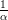be the zeroes of P(x).
P(a) = ax2 + bx + c …(given in the question)
Product of zeroes =⇒ α ×=⇒ 1 =⇒ a = c
Coefficient of x2 = Constant term

Form a quadratic polynomial whose zeroes are 3 + √2 and 3 – √2. (2012)

જવાબ : Sum of zeroes = (3 + √2) + (3 – √2) = 6
Product of zeroes = (3 + √2) x (3 – √2) = (3)2 – (√2)2 = 9 – 2 = 7
Quadratic polynomial = x2 – 6x + 7

Find a quadratic polynomial, the stun and product of whose zeroes are √3 and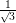respectively. (2014)

જવાબ : Sum of zeroes = √3
Product of zeroes =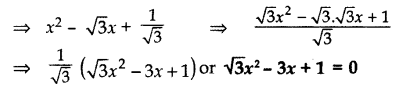Find a quadratic polynomial, the sum and product of whose zeroes are 0 and -√2 respectively. (2015)

x2 – (Sum of zeroes) x + (Product of zeroes)
= x2 – (0)x + (-√2)
= x2 – √2

Find the zeroes of the quadratic polynomial √3 x2 – 8x + 4√3. (2013)

જવાબ :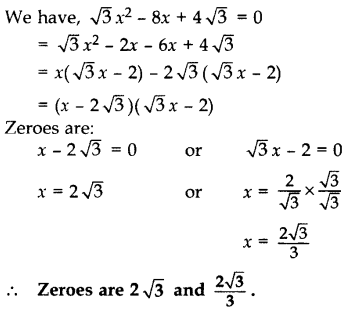If the zeroes of the polynomial x2 + px + q are double in value to the zeroes of 2x2 – 5x – 3, find the value of p and q. (2012)

જવાબ : We have, 2x2 – 5x – 3 = 0
= 2x2 – 6x + x – 3
= 2x(x – 3) + 1(x – 3)
= (x – 3) (2x + 1)
Zeroes are:
x – 3 = 0 or 2x + 1 = 0
⇒ x = 3 or x =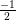Since the zeroes of required polynomial is double of given polynomial.
Zeroes of the required polynomial are:
3 × 2, (× 2), i.e., 6, -1
Sum of zeroes, S = 6 + (-1) = 5
Product of zeroes, P = 6 × (-1) = -6
Quadratic polynomial is x2 – Sx + P
⇒ x2 – 5x – 6 …(a)
Comparing (a) with x2 + px + q
p = -5, q = -6

Can (x – 2) be the remainder on division of a polynomial p(x) by (2x + 3)? Justify your answer. (2016 OD)

જવાબ : In case of division of a polynomial by another polynomial, the degree of the remainder (polynomial) is always less than that of the divisor. (x – 2) can not be the remainder when p(x) is divided by (2x + 3) as the degree is the same.

Find a quadratic polynomial whose zeroes are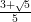and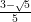. (2013)

જવાબ :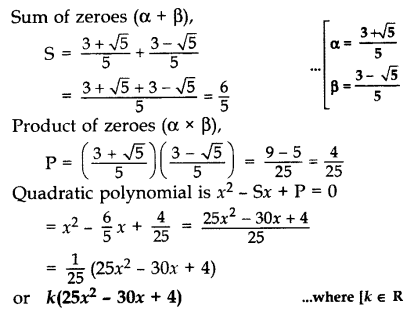= a(25x2 – 30x + 4 )                                           … where [a є R

Find the quadratic polynomial whose zeroes are -2 and -5. Verify the relationship between zeroes and coefficients of the polynomial. (2013)

જવાબ : Sum of zeroes = (-2) + (-5) = -7
Product of zeroes = (-2)(-5) = 10
Quadratic polynomial = x2 – (-7)x + 10
= x2 + 7x + 10
Verification:
Here a = 1, b = 7, c = 10
Sum of zeroes = (-2) + (-5) = 7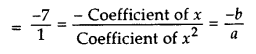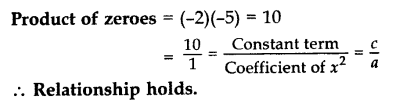Find the zeroes of the quadratic polynomial 3x2 – 75 and verify the relationship between the zeroes and the coefficients. (2014)

જવાબ : We have, 3x2 – 75
= 3(x2 – 25)
= 3(x2 – 52)
= 3(x – 5)(x + 5)
Zeroes are:
x – 5 = 0 or x + 5 = 0
x = 5 or x = -5 ------(1)
Verification:
Here a = 3, b = 0, c = -75
Sum of the zeroes = 5 + (-5) = 0       [from eq.1]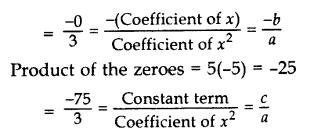Find the zeroes of p(x) = 2x2 – x – 6 and verify the relationship of zeroes with these co-efficients. (2017 OD)

જવાબ : p(x) = 2x2 – x – 6 …[Given in question]
= 2x2 – 4x + 3x – 6
= 2x (x – 2) + 3 (x – 2)
= (x – 2) (2x + 3)
Zeroes are:
x – 2 = 0 or 2x + 3 = 0
x = 2 or x =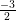Verification:
Here a = 2, b = -1, c = -6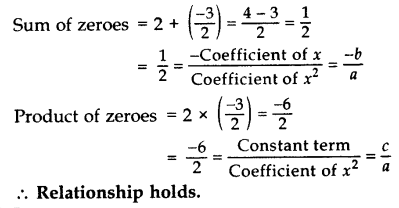What must be subtracted from the polynomial f(x) = x4 + 2x3 – 13x2 – 12x + 21 so that the resulting polynomial is exactly divisible by x2 – 4x + 3? (2012, 2017 D)

જવાબ :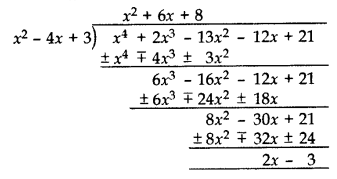(2x – 3) should be subtracted from x4 + 2x3 – 13x2 – 12x + 21 as it is the remainder

Find a quadratic polynomial, the sum and product of whose zeroes are -8 and 12 respectively. Hence find the zeroes. (2014)

જવાબ : Let Sum of zeroes (α + β) = S = -8 …[Given in question]
Product of zeroes (αβ) = P = 12 …[Given in question]
Quadratic polynomial is x2 – Sx + P
= x2 – (-8)x + 12
= x2 + 8x + 12
= x2 + 6x + 2x + 12
= x(x + 6) + 2(x + 6)
= (x + 2)(x + 6)
Zeroes are:
x + 2 = 0 or x + 6 = 0
x = -2 or x = -6

Find a quadratic polynomial, the sum and product of whose zeroes are 0 and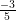respectively. Hence find the zeroes. (2015)

જવાબ : Quadratic polynomial = x2 – Sx + P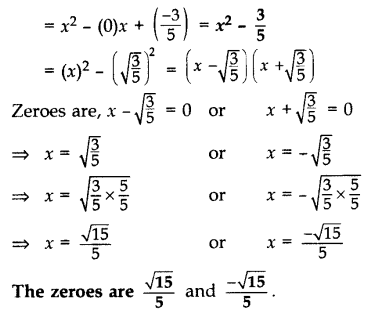​​​​​​​

Divide 3x2 + 5x – 1 by x + 2 and verify the division algorithm. (2013 OD)

જવાબ :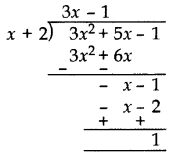Remainder = 1
Quotient = (3x – 1)
Verification:
Divisor × Quotient + Remainder
= (x + 2) × (3x – 1) + 1
= 3x– x + 6x – 2 + 1
= 3x2 + 5x – 1
= Dividend

If sum of the squares of zeroes of the quadratic polynomial 6x2 + x + l is 25/36, the value of l is

જવાબ : -2

The value of k for which the polynomial x3 + 4x2 –kx + 8 is exactly divisible by (x – 2) is

જવાબ : 16

If one of the zeroes of the quadratic polynomial (k – 1)x2 + mx + 1 is –3, then the value of m is

જવાબ : 4/3

If one of the zeroes of the cubic polynomial x3 + ax2 + bx + c is –1, then the

Product of the other two zeroes is

જવાબ : b – a + 1

Given that one of the zeroes of the cubic polynomial ax3 + bx2 + cx + d is zero, the product of the other two zeroes is

જવાબ : c/a

If the zeroes of the quadratic polynomial x2 + (a + 1)x + b are 2 and –3, then a&b ?

જવાબ : a = 0, b = – 6

The degree of the polynomial (x + 1)(x2 – x – x4 +1) is:

જવાબ : 3

The number of polynomials having zeroes as –2 and 5 is ______________

જવાબ : Infinite

Sign of the zeroes of the quadratic polynomial x2 + 99x + 127 are

જવાબ : Both Negative

If (x - 2) and [x - ½ ] are the factors of the polynomials qx2 + 5x + r prove that q = r

જવાબ : 4q+10+r=0 -----(a)
q/4 +5/2 +r=0 or q+10+4r=0 -----(b)
Subtracting a from b
3q-3r=0
q=r

If the zeroes of the quadratic polynomial ax2 + bx + c, c ≠ 0 are equal, then which constant should have same signs?

જવાબ : a & c

A cubic polynomial is given below
S(x) =x3 -3x2+x+1
The zeroes of the polynomial are given as (a-b) ,a  and (a+b). What is the value a and b

જવાબ : a=1,b=√2 or -√2

p(x) = x4 -6x3 +16x2 -25x +10
q(x) = x2-2x+k
It is given
p(x) = r(x) q(x) + (x+m)
Find the value of k and m

જવાબ : K=5 and a=-5

If the zeroes of the quadratic equation are 11 and 2 ,what is expression for quadratic?

જવાબ : (x-11)(x-2)

Z(x) = px2+(p-2)x +2. If  2 is the zero of this polynomial,what is the value of p

જવાબ : -1/2

If p and q are the zeroes of the polynomial  x2-11x +30, Find the value of p3 + b3

જવાબ : 341

Find the remainder  when x4+x3-2x2+x is divided by x-1

જવાબ : 1

Factorize the following : 1+8x3

જવાબ : (2x+1)(4x2-2x+1)

Factorize the following : x3-23x2+142x-120

જવાબ : (x-1)(x-10)(x-12)

Factorize the following : 3x3 -x2-3x+1

જવાબ : (3x-1)(x-1)(x+1)

Factorize the following : x2 +9x+18

જવાબ : (x+6)(x+3)

State whether the statement is true or  false :- Graph of constant polynomial never meets x axis

જવાબ : True

State whether the statement is true or  false :- Graph of polynomial (x2-1) meets the  x-axis at one point

જવાબ : False

State whether the statement is true or  false :- The degree of zero polynomial is not defined

જવાબ : True

State whether the statement is true or  false :- 1,2 are the zeroes of x2-3x+2

જવાબ : True

State whether the statement is true or  false :- A binomial may have degree 6

જવાબ : True

State whether the statement is true or  false :- Every real number is the zero’s of zero polynomial

જવાબ : True

State whether the statement is true or  false :- Every linear polynomial has only one zero

જવાબ : True

State whether the statement is true or  false :- The factor of 3x2 -x-4 are  (x+1)(3x-4)

જવાબ : True

State whether the statement is true or  false :- P(x) =x-1 and g(x) =x2-2x +1 .  p(x) is a factor of g(x)

જવાબ : True

Divide 4x3 + 2x2 + 5x – 6 by 2x2 + 1 + 3x and verify the division algorithm. (2013)

જવાબ :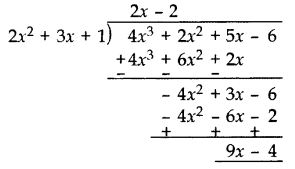Divisor [D]= (2x2 + 3x + 1)

Quotient [Q] = (2x – 2)
Remainder [R] = (9x – 4)
Verification:
[D] × [Q] + [R]
= (2x2 + 3x + 1) × (2x – 2) + 9x – 4
= 4x3 – 4x2 + 6x2 – 6x + 2x – 2 + 9x – 4
= 4x3 + 2x2 + 5x – 6
= Dividend

Given that x – √5 is a factor of the polynomial x3 – 3√5 x2 – 5x + 15√5, find all the zeroes of the polynomial. (2012, 2016)

જવાબ : Let,the polynomial  P(x) = x3 – 3√5 x2 – 5x + 15√5
x – √5 is a factor of the given polynomial.
Put x = -√5,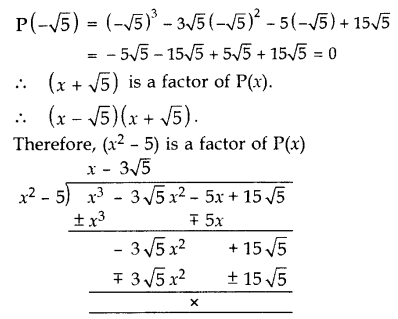Another zero:
x – 3√5 = 0 x = 3√5
All the zeroes of P(x) are -√5, √5 and 3√5.

If a polynomial x4 + 5x3 + 4x2 – 10x – 12 has two zeroes as -2 and -3, then find the other zeroes. (2014)

જવાબ : Given two zeroes are -2 and -3.
(x + 2)(x + 3) = x2 + 3x + 2x + 6 = x2 + 5x + 6
Dividing the given equation with x2 + 5x + 6, we get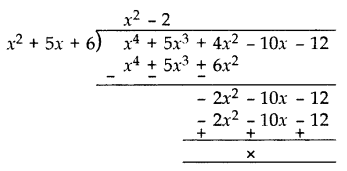x4 + 5x+ 4x2 – 10x – 12
= (x2 + 5x + 6)(x2 – 2)
= (x + 2)(x + 3)(x – √2 )(x + √2 )
Other zeroes are:
x – √2 = 0 or x + √2 = 0
x = √2 or x = -√2

Find all the zeroes of the polynomial 8x4 + 8x3 – 18x2 – 20x – 5, if it is given that two of its zeroes are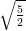and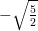. (2014, 2016 D)

જવાબ :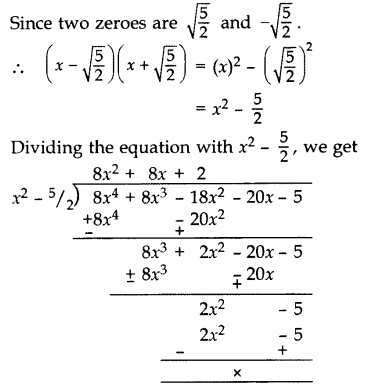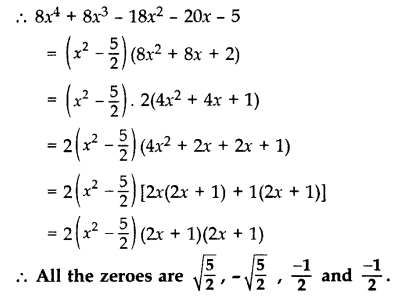If p(x) = x3 – 2x2 + mx + 5 is divided by (x – 2), the remainder is 11. Find m. Hence find all the zeroes of x3 + mx2 + 3x + 1.

જવાબ : p(x) = x3 – 2x2 + mx + 5,
When x – 2,
p(2) = (2)3 – 2(2)2 + m(2) + 5
11 = 8 – 8 + 2m + 5
11 – 5 = 2m
6 = 2m
m = 3
Let q(x) = x3 + mx2 + 3x + 1
= x3 + 3x2 + 3x + 1
= x3 + 1 + 3x2 + 3x
= (x)3 + (1)3 + 3x(x + 1)
= (x + 1)3
= (x + 1) (x + 1) (x + 1) …[ a3 + b3 + 3ab (a + b) = (a + b)3]
All zeroes are:
x + 1 = 0 x = -1
x + 1 = 0 x = -1
x + 1 = 0 x = -1
Hence zeroes are -1, -1 and -1.

If α and β are zeroes of p(x) = kx2 + 4x + 4, such that α2 + β2 = 24, find k. (2013)

જવાબ : We have, p(x) = kx2 + 4x + 4
Here a = k, b = 4, c = 4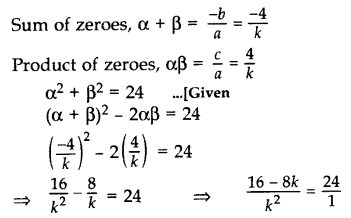24k2 = 16 – 8k
24k2 + 8k – 16 = 0
3k2 + k – 2 = 0 …[Dividing equation by 8]
3k2 + 3k – 2k – 2 = 0
3k(k + 1) – 2(k + 1) = 0
(k + 1)(3k – 2) = 0
k + 1 = 0 or 3k – 2 = 0
k = -1 or k =If α and β are the zeroes of the polynomial p(x) = 2x2 + 5x + k, satisfying the relation, α2 + β2 + αβ =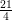then find the value of k. (2017 OD)

જવાબ : Given , p(x) = 2x2 + 5x + k
Here a = 2, b = 5, c = k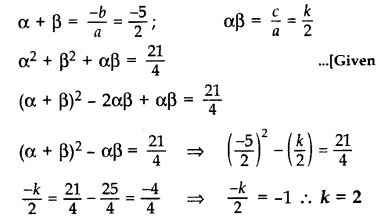What must be subtracted from p(x) = 8x4 + 14x3 – 2x2 + 8x – 12 so that 4x2 + 3x – 2 is factor of p(x

જવાબ :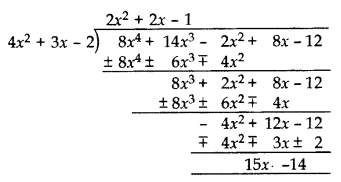Remainder = (15x – 14).

Hence, Polynomial to be subtracted by (15x – 14).

Find the values of a and b so that x4 + x3 + 8x2 +ax – b is divisible by x2 + 1. (2015)

જવાબ :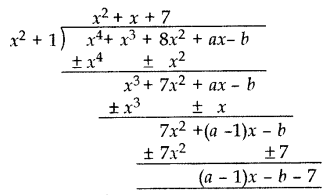If x4 + x3 + 8x2 + ax – b is divisible by x2 + 1
=> Remainder = 0
(a – 1)x – b – 7 = 0
(a – 1)x + (-b – 7) = 0 . x + 0
a – 1 = 0, -b – 7 = 0
a = 1, b = -7
a = 1, b = -7

If a polynomial 3x4 – 4x3 – 16x2 + 15x + 14 is divided by another polynomial x2 – 4, the remainder comes out to be px + q. Find the value of p and q. (2014)

જવાબ :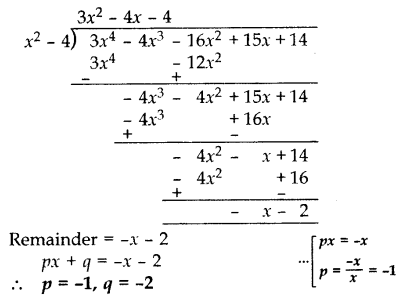If the polynomial (x4 + 2x3 + 8x2 + 12x + 18) is divided by another polynomial (x2 + 5), the remainder comes out to be (ax +b ), find the values of a and b.

જવાબ :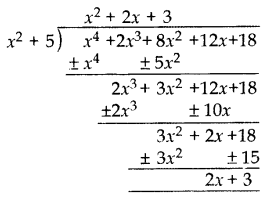Remainder = 2x + 3
ax + b = 2x + 3
a = 2 and b = 3

Find the condition that zeroes of polynomial p(x) = ax2 + bx + c are reciprocal of each other. (2017 OD)

જવાબ : Let s and 1/ s be the zeroes of P(x).
P(x) = ax2 + bx + c …(given in question)
Product of zeroes = c/a
s × 1/s = c/a
1 = c/a

a = c (Required condition)
Coefficient of x2 = Constant term

Form a quadratic polynomial whose zeroes are 3 + √2 and 3 – √2. (2012)

જવાબ : Sum of zeroes = (3 + √2) + (3 – √2) = 6
Product of zeroes = (3 + √2) x (3 – √2) = (3)2 – (√2)2 = 9 – 2 = 7
Quadratic polynomial = x2 – 6x + 7

Find a quadratic polynomial, the sum and product of whose zeroes are √3 and 1/√3 respectively. (2014)

જવાબ : Sum of zeroes = √3
Product of zeroes = 1/√3

Quadratic polynomial = x2 – Sx + P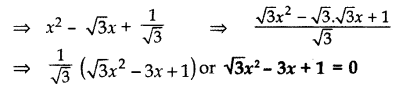Find the zeroes of p(x) = 2x2 – x – 6 and verify the relationship of zeroes with these co-efficients. (2017 OD)

જવાબ : p(x) = 2x2 – x – 6 …[Given in question]
= 2x2 – 4x + 3x – 6
= 2x (x – 2) + 3 (x – 2)
= (x – 2) (2x + 3)
Zeroes are:
x – 2 = 0 or 2x + 3 = 0
x = 2 or x = -3 / 2
Verification:
Here a = 2, b = -1, c = -6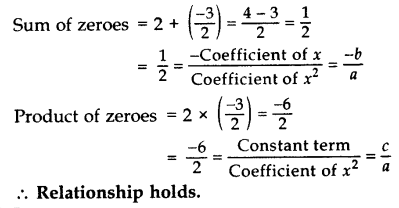​​​​​​​

If the zeroes of the polynomial x2 + px + q are double in value to the zeroes of 2x2 – 5x – 3, find the value of p and q. (2012)

જવાબ : We have, 2x2 – 5x – 3 = 0
= 2x2 – 6x + x – 3
= 2x(x – 3) + 1(x – 3)
= (x – 3) (2x + 1)
Zeroes are:
x – 3 = 0 or 2x + 1 = 0
x = 3 or x =  -1 /2

Since the zeroes of required polynomial is double of given polynomial.
Zeroes of the required polynomial are:
3 × 2, -1/2× 2, i.e., 6, -1
Sum of zeroes, S = 6 + (-1) = 5
Product of zeroes, P = 6 × (-1) = -6
Quadratic polynomial is x2 – Sx + P
x2 – 5x – 6 …(A)
Comparing (A) with x2 + px + q
p = -5, q = -6

Find the zeroes of the quadratic polynomial √3 x2 – 8x + 4√3. (2013)

જવાબ : Given equation, √3 x2 – 8x + 4√3=0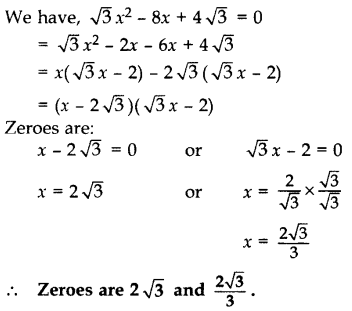Find a quadratic polynomial whose zeroes are (3+5)/5  & (3-5)/5. (2013)

જવાબ :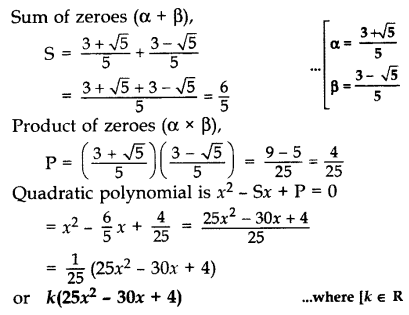Find the quadratic polynomial whose zeroes are -2 and -5. Verify the relationship between zeroes and coefficients of the polynomial. (2013)

જવાબ : Sum of zeroes = (-2) + (-5) = -7
Product of zeroes = (-2)(-5) = 10
Quadratic polynomial is x2 – Sx + P = 0
= x2 – (-7)x + 10
= x2 + 7x + 10
Verification:
Here a = 1, b = 7, c = 10
Sum of zeroes = (-2) + (-5) = 7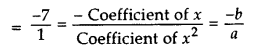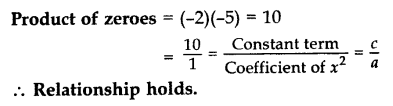​​​​​​​

Find the zeroes of the quadratic polynomial 3x2 – 75 and verify the relationship between the zeroes and the coefficients. (2014)

જવાબ : 3x2 – 75
= 3(x2 – 25)
= 3(x2 – 52)
= 3(x – 5)(x + 5)
Zeroes are x – 5 = 0 or x + 5 = 0
x = 5 or x = -5
Verification:
Here a = 3, b = 0, c = -75
Sum of the zeroes = 5 + (-5) = 0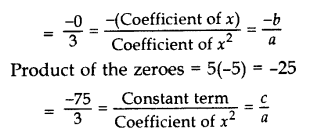​​​​​​​

### There are No Content Availble For this Chapter

Degree of polynomial

 1 x5 +x -25 A 0 2 (x+1)(x3 + x2 + 31) B 2 3 9 C 5 4 x2+1 D 4

જવાબ :

1-C, 2-D, 3-A, 4-B

Equations and Root

 1 X2+ 10x + 25 A -5 & 5 2 X2-5x-50 B 5 & 5 3 X2 -25 C -2 & 20 4 X2+18x-40 D -10 & 5

જવાબ :

1-B, 2-D, 3-A, 4-C

Equations and Root

 1 X2+ 31x + 30 A -20 & -8 2 X2-28x+160 B -7 & 7 3 X2 -49 C 2 & 3 4 X2+5x+ 6 D 1 & 30

જવાબ :

1-D, 2-A, 3-B, 4-C

Degree of polynomial

 1 ( X2 +x -25) / (x+1) A 6 2 (x+1)(x5 + x2 + 31) B 1 3 X49/x7 C 10 4 (x2+1)5 D 42

જવાબ :

1-B, 2-A, 3-D, 4-C

Equations and Root

 1 X2 + 16x + 60 A -20 & 4 2 X2 + 16x – 80 B 6 & 10 3 X2 -16x -80 C 8 & 10 4 X2 + 18x + 80 D 20 & -4

જવાબ :

1-B, 2-D, 3-A, 4-C

P(x)=5x3 -3x2+7x+1

 1 P(1) A 1 2 P(0) B 10 3 P(-1) C -65 4 P(-2) D -14

જવાબ :

1-B, 2-A, 3-D,4-D

Equations and Root

 1 X2 + 12x + 36 A -6 & -6 2 X2 + 12x – 160 B 6 & 6 3 X2 -12x + 36 C -14 & 2 4 X2 -12x – 28 D 20 & -8

જવાબ :

1-B, 2-D, 3-A, 4-C

Equations and Root

 1 X2 + 17x + 30 A 18 & -1 2 X2 + 17x – 18 B 15 & 2 3 X2 -17x + 16 C -14 & -3 4 X2 -17x + 42 D -16 & -1

જવાબ :

1-B, 2-A, 3-D, 4-C

Equations and Root

 1 X2 + 11x + 10 A -12 & 1 2 X2 + 11x + 18 B -13 & 2 3 X2 -11x -12 C 10 & 1 4 X2 -11x - 26 D 9 & 2

જવાબ :

1-C, 2-D, 3-A, 4-B

Equations and Root

 1 X2 + 10x + 25 A 15 & -5 2 X2 + 10x - 45 B 5 & 5 3 X2 -10x - 96 C -14 & -3 4 X2 -10x + 25 D -5 & -5

જવાબ :

1-B, 2-A, 3-D,4-D